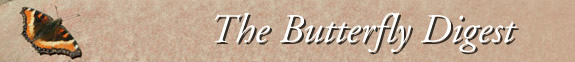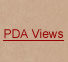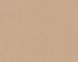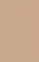Jump to : Today March 22, 2019 March 21, 2019 March 20, 2019 March 19, 2019 March 18, 2019 March 17, 2019 March 16, 2019 March 15, 2019 March 14, 2019 March 13, 2019 March 12, 2019 March 11, 2019 March 10, 2019 March 9, 2019 March 8, 2019 March 7, 2019 March 6, 2019 March 5, 2019 March 4, 2019 March 3, 2019 March 2, 2019 March 1, 2019 February 28, 2019 February 27, 2019 February 26, 2019 February 25, 2019 February 24, 2019 February 23, 2019 February 22, 2019 February 21, 2019     |    View All Lists    |    FAQ

Thanks Jim Great Information its so hard to sort out what all =is in the group but the area i was in they were lots of Painted ladies stop=ping to sip nectar Massive blooms and lots of new looking Painted ladies si=pping on Probably every flower that was there fiddle necks, mustard , man r=oot or wild cucumber invasive daisies the hills and flats are covered in bl=ooms And yes they are stopping to eat and yes they fly fast! after a snack!=

=20=20=20=20=20=20=20=20
=20=20=20=20=20=20=20=20=20=20=20=20=20=20=20=20
On Wednesday, March 13, 2019, 8:40:47 AM PDT, Jim Brock= [SoWestLep] <> w=rote:

=20=20=20=20=20=20=20=20=20=20=20=20

Robert,

There might be some literature on this subject. I have personally seen= Red Admiral's and Common buckeyes flying with the cardui movement in the V=irgin Mountains of Arizona.

My question is, does anybody know if anybody is looking at the reverse= migration of cardui in the fall in North America? I suspect there could be= a less massive return of cardui to some points south (Mexico?) like they d=o in Europe where they head south from Scandinavia to northern Africa.

Jim B

-----Original Message-----
From: ROBERT GORMAN [SoWestLep] <SoWestLep-noreply@=yahoogroups.com>
To: SoWestLep <>
Sent: Tue, Mar 12, 2019 7:00 pm
Subject: Re: [SoWestLep] Painted Lady Migration

=20=20
=20=20=20=20
=20=20=20=20=20=20=20=20=20=20=20=20=20=20=20=20=20=20
=20=20=20=20=20=20=20=20
Paul, Thousands or Probably hundreds of thousands today going through =Orange County today. I Could count on shrubs by fives and Tens that there w=ere more than fifty getting a Drink at a time. Clouds nonstop for several h=ours starting around nine with peak at about 2pm. Do you or anybody out the=re know how far they go when they migrate. Also it seems as the West Coast =Ladies, Red Admirals, American Ladies Were absent today at local hilltops W=onder Why. Do they Get Caught up in all this and go north also? I have read= that at times one in fifty or a Hundred is said to be a Tortoise Shell? Th=anks Paul, Bob

=20=20=20=20=20=20=20=20
=20=20=20=20=20=20=20=20=20=20=20=20
=20=20=20=20=20=20=20=20=20=20=20=20=20=20=20=20=20=20=20=20=20=20=20=20=20=20=20=20=20=20=20=20
On Monday, March 11, 2019, 7:07:03 PM PDT, Paul Russell= <> wrote:
=20=20=20=20=20=20=20=20=20=20=20=20=20=20=20=20

=20=20=20=20=20=20=20=20=20=20=20=20=20=20=20=20

=20=20=20=20=20=20=20=20=20=20=20=20=20=20=20=20
Thanks, still light here, but it has been cold with clouds, wind and o=ccasional rain.
Paul

On Mar 11, =2019, at 3:52 PM, ROBERT GORMAN <" =rel=3D"nofollow" target=3D"_blank">> wrote:
=20=20=20=20=20=20=20=20
Paul, out i=n Borrego Desert Near Corizo Wash on the S-2 hwy near Ocotillo California a=t times there were 30 Plus at one time passing us making us almost Dizzy tr=ying to walk, lots of larva on Lupin of Panted ladies, also Huge White Line=d Sphinx larva. The Largest I have ever yet seen Mowing the flowers down, t=his super bloom may be over in lower elevations by this coming weekend. the= Washes and Hill sides are Blooming Mountain Palm Springs in Borrego Desert= Seemed like it avoided the frost and snows of other area's was more Shelte=red an lots more flying. Warm and sunny even on sunday the Tenth when it wa=s said to be cloudy!

=20=20=20=20=20=20=20=20
=20=20=20=20=20=20=20=20=20=20=20=20
=20=20=20=20=20=20=20=20=20=20=20=20=20=20=20=20=20=20=20=20=20=20=20=20=20=20=20=20=20=20=20=20
On Thursday, March 7, 2019, 8:10:54 PM PST, Paul Russel=l " rel=3D"nofollow" target=3D"_blank">= [SoWestLep] <SoWestLep-noreply@yahoo=groups.com> wrote:
=20=20=20=20=20=20=20=20=20=20=20=20=20=20=20=20

=20=20=20=20=20=20=20=20=20=20=20=20=20=20=20=20

=20=20=20=20=20=20=20=20=20=20=20=20=20=20=20=20

=20=20
=20=20=20=20
Today (Marc=h 7) in Santa Barbara we observed what may be the vanguard of a mass migrat=ion of Painted Ladies, all heading west. Numbers were several per minute, =which is still light, but we hadn=E2=80=99t seen even a singleton for some =time. Have others to the east of us seen this, and how abundant are they?<=br clear=3D"none" class=3D"ydpc351fdd7yiv1616227316ydpe3f3ea1fyiv9033642059=">Paul Russell
" rel=3D"nofollow" target=3D"_blank">ma=

=20=20=20=20=20=20=20=20=20=20=20=20=20

=20=20=20=20=20=20=20=20=20=20=20=20=20
=20=20=20=20=20=20=20=20=20

__._,_.___
=20=20=20=20=20=20=20=20=20=20=20=20

Posted by: ROBERT GORMAN <>
<=br>=20=20=20=20
=20=20=20=20=20=20=20=20=20
=20=20=20
__,_._,___
------=_Part_4889361_18509664.1552496470300--